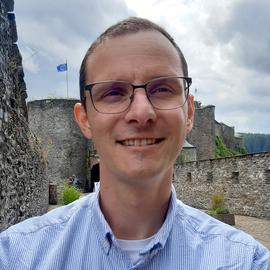# The importance of phase in complex compressive sensing

Date
Sep 10, 2021
Location
Institut Pascal, Paris-Saclay University (France)

(joint work with T. Feuillen)

Abstract: In this talk, we consider the estimation of a sparse (or low-complexity) signal from the phase of complex random measurements, a “phase-only compressive sensing” (PO-CS) scenario. This study is motivated by the fixed range of such measurements, which can for instance ease further data quantization methods, with potential applications in radar, computed tomography or computational imaging. With high probability and up to a global unknown amplitude, we show that perfect signal recovery is possible if the sensing matrix is a complex Gaussian random matrix and the number of measurements is large compared to the signal sparsity. Our approach consists in recasting the (non-linear) PO-CS scheme as a linear compressive sensing model. We built it from a signal normalization constraint and a phase-consistency constraint. Practically, we achieve stable and robust signal direction estimation from the basis pursuit denoising program. Numerically, robust signal direction estimation is reached at about twice the number of measurements needed for signal recovery in compressive sensing